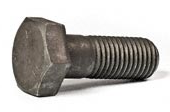Engineering ToolBox - Resources, Tools and Basic Information for Engineering and Design of Technical Applications!

# Bolt Stretching and Tensile Stress

## Tensile stress and Hooke's Law.### Elongation

Bolt stretching according Hook's Law can be calculated as

dl = F L / E A                      (1)

where

dl = change in length of bolt (inches, m)

F = applied tensile load (lb, N)

L = effective length of bolt where tensile strength is applied (inches, m)

E = Young's Modulus of Elasticity (psi, N/m2)

A = tensile stress area of the bolt (square inches, m2)

### Stress Area

The tensile stress area can be calculated as

A = (π / 4) (d - 0.9743 / n)2                       (2)

where

d = nominal diameter of bolt (in)

n = 1 / p = number of threads per inch

p = pitch, length per thread (in)

### Tensile Stress

Tensile stress can be calculated as

σ = F / A                      (3)

where

σ = tensile stress (psi, N/m2 (Pa))

### Example - Bolt Stretching - Imperial Units

• stud diameter : 7/8 inches
• Young's Modulus steel : 30 106 psi
• designed bolt load : 10000 lb
• effective length : 5 inches

The tensile stress area can be calculated as

A = 0.7854 ((7/8 in) - 0.9743 / 9)2

= 0.46 (in2)

The elongation can be calculated as

dl = (10000 lb) (5 in) / ((30 106 psi) (0.46 in2))

= 0.0036 (inches)

The tensile stress can be calculated as

σ = (10000 lb) / (0.46 in2)

= 21740 psi

## Related Documents

• ### ASME/ANSI B16 Standards for Pipes and Fittings

The ASME B16 standards covers pipes and fittings in cast iron , cast bronze, wrought copper and steel.
• ### Bolt Torque Calculator

Calculate required bolt torque.
• ### Bronze Flanges - ASME/ANSI 300 lb

Flange diameters, thickness, bolt circles, numbers and diameter of bolts for ASME/ANSI B16.15 - Cast Bronze Threaded Fittings - 300 lb Bronze - Flanges with plain faces.
• ### Cast Iron Flanges - Stud Reference Chart

The number, diameter and length of studs for cast iron flanges class 125 and 250.
• ### Hooke's Law

Hooke's law - force, elongation and spring constant.
• ### ISO 724 - Metric Threads

Dimensions of metric threads according ISO 724.
• ### Metals and Alloys - Young's Modulus of Elasticity

Elastic properties and Young's modulus for metals and alloys like cast iron, carbon steel and more.
• ### Metric Bolt Heads and Wrench Sizes

ANSI/SO, DIN and JIS bolts.
• ### Metric Bolts - Minimum Ultimate Tensile and Proof Loads

Minimum ultimate tensile and proof loads for metric bolts with coarse or fine threads.
• ### Metric Steel Bolts - Grades and Property Classes

Metric steel bolts, screws and studs - proof and tensile strength.
• ### Modulus of Rigidity

Shear Modulus (Modulus of Rigidity) is the elasticity coefficient for shearing or torsion force.
• ### Pipe Class Ratings vs. Pressure Numbers (PN)

Pressure numbers (PN) vs. flange class designations.
• ### Pipe Flanges and Gaskets - Dimensions

Gasket dimensions according ASME B16.5 Pipe Flanges and Flange Fittings.
• ### Reduced Torque with Lubricated Bolts

Lubrication effect on bolt tension and torque.
• ### Steel Bolts - ASTM Grades

ASTM steel bolts - proof and tensile strength.
• ### Steel Bolts - SAE Grades

SAE steel bolts - grades ranging grade 1 to 8.2 - proof and tensile strength.

• ### UNC and UNF - Unified Inch Screw Threads

ANSI/ASME B1.1 Unified National Thread - UNC Coarse series and UNF Fine series.
• ### US Bolts - Tensile Strength and Proof Loads

Tensile strength and proof loads SAE bolts.
• ### US Hex Bolts - Inches

ANSI/ASME B18.2.1 Dimensions of Hex Bolts - Imperial units.
• ### US Hex Bolts - Metric

ANSI/ASME B18.2.3M Dimensions of Hex Bolts - Metric units.
• ### Wrench Spanners - Inches vs. mm

Convert between US Inches and metric mm wrench and spanner sizes.
• ### Young's Modulus, Tensile Strength and Yield Strength Values for some Materials

Young's Modulus (or Tensile Modulus alt. Modulus of Elasticity) and Ultimate Tensile Strength and Yield Strength for materials like steel, glass, wood and many more.

## Engineering ToolBox - SketchUp Extension - Online 3D modeling!

Add standard and customized parametric components - like flange beams, lumbers, piping, stairs and more - to your Sketchup model with the Engineering ToolBox - SketchUp Extension - enabled for use with older versions of the amazing SketchUp Make and the newer "up to date" SketchUp Pro . Add the Engineering ToolBox extension to your SketchUp Make/Pro from the Extension Warehouse !

We don't collect information from our users. More about

## Citation

• The Engineering ToolBox (2008). Bolt Stretching and Tensile Stress. [online] Available at: https://www.engineeringtoolbox.com/bolt-stretching-d_1164.html [Accessed Day Month Year].

Modify the access date according your visit.

9.19.12